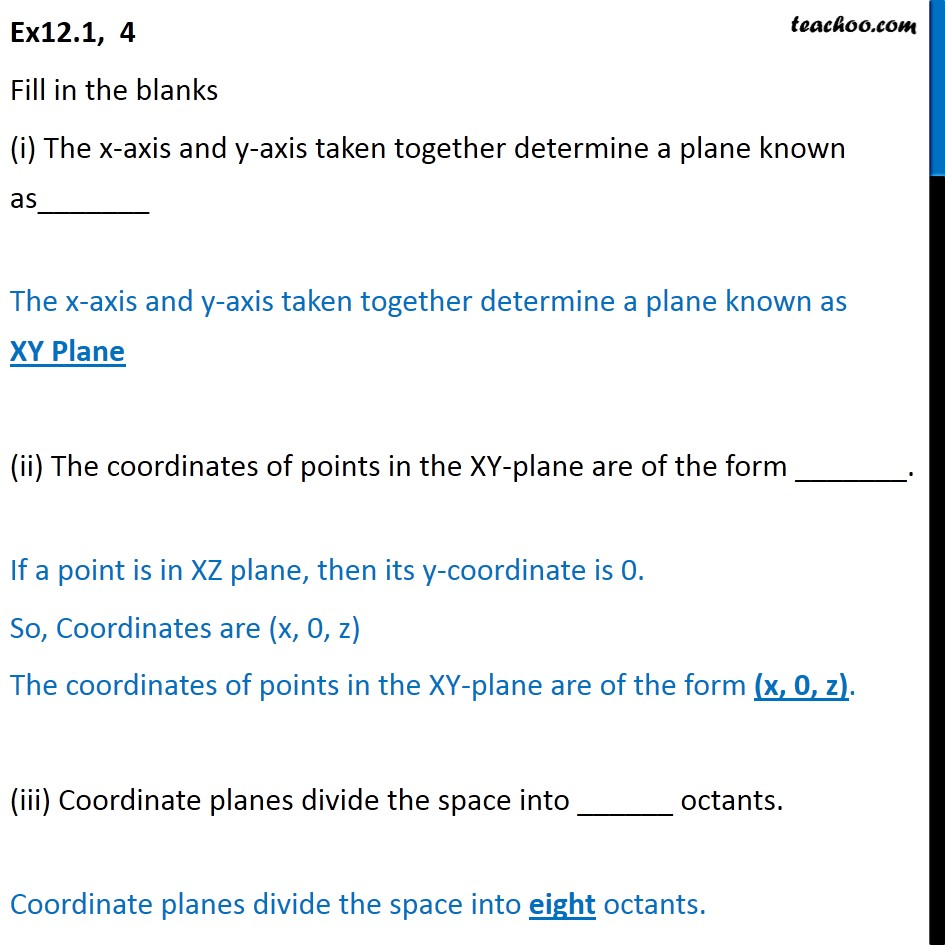1. Chapter 12 Class 11 Introduction to Three Dimensional Geometry
2. Serial order wise
3. Ex 12.1

Transcript

Ex12.1, 4 Fill in the blanks (ii) The x-axis and y-axis taken together determine a plane known as_______ The x-axis and y-axis taken together determine a plane known as XY Plane (i) The coordinates of points in the XY-plane are of the form _______. If a point is in XZ plane, then its y-coordinate is 0. So, Coordinates are (x, 0, z) The coordinates of points in the XY-plane are of the form (x, 0, z). (iii) Coordinate planes divide the space into ______ octants. Coordinate planes divide the space into eight octants.

Ex 12.1